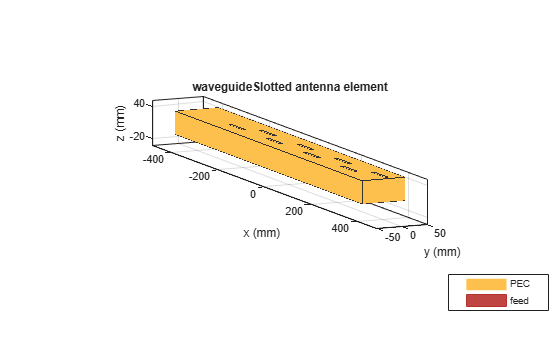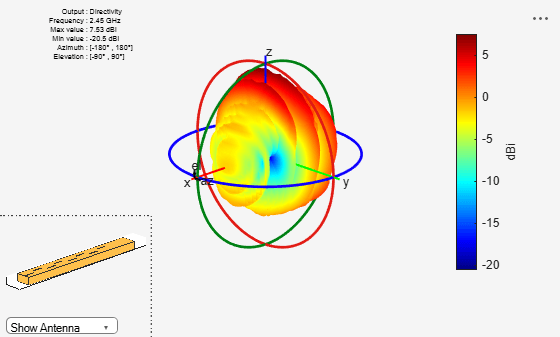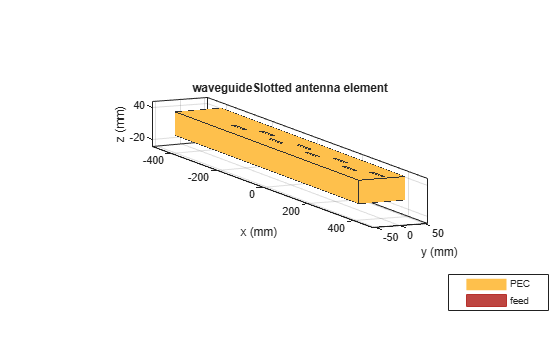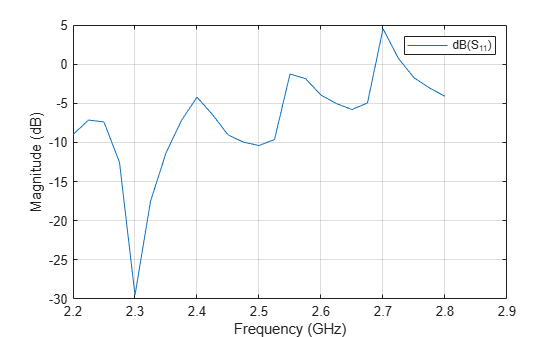Documentation

# waveguideSlotted

Create slotted waveguide antenna

## Description

The `waveguideSlotted` object creates a slotted waveguide antenna. There are different types of slotted waveguides, including longitudinal slots, transversal slots, center inclined slots, inclined slots, and inclined slots cut into a narrow wall. Slotted waveguide antennas are used in navigation radar as an array fed by a waveguide.## Creation

### Syntax

``ant = waveguideSlotted``
``ant = waveguideSlotted(Name,Value)``

### Description

example

````ant = waveguideSlotted` creates a slotted waveguide antenna on the X-Y plane. The circumference of the antenna is chosen for an operating frequency of 2.45 GHz.```

example

````ant = waveguideSlotted(Name,Value)` sets properties using one or more name-value pairs. For example, `ant = waveguideSlotted('Height',1)` creates a slotted waveguide with a height of 1 meter.```

### Output Arguments

expand all

Slotted waveguide antenna, returned as a `waveguideSlotted` object.

## Properties

expand all

Length of the waveguide (n times lambda), specified as a real-valued scalar in meters. n is the number of slots in the waveguide.Example: `'Length',0.760`

Example: `ant.Length = 0.760`

Data Types: `double`

Width of the waveguide (a), specified as a real-valued scalar in meters.Example: `'Width',0.0840`

Example: `ant.Width = 0.0840`

Data Types: `double`

Height of the waveguide (b), specified as a real-valued scalar in meters. Please see image in `Width` property.

Example: `'Height',0.0340`

Example: `ant.Height = 0.0340`

Data Types: `double`

Number of slots (n), specified as a scalar integer.

Example: `'Numslots',7`

Example: `ant.Numslots = 7`

Data Types: `double`

Shape of waveguide slot, specified as one of the following objects: `antenna.Circle`, `antenna.Polygon`, `antenna.Rectangle`.

Example: `'Slot',antenna.rectangle['Length',0.035]`

Example: ```ant.Slot = antenna.rectangle['Length',0.035]```

Data Types: `double`

Distance from the closed face edge to the top slot center, specified as a real-valued scalar in meters.

Example: `'SlotToTop',0.0503`

Example: `ant.SlotToTop = 0.0503`

Data Types: `double`

Space between the centers of two adjacent slots, specified as a real-valued scalar in meters.

Example: `'SlotSpacing',0.0906`

Example: `ant.SlotSpacing = 0.0906`

Data Types: `double`

Slot displacement from the centreline of the width of the waveguide to the center of the slot, specified as a real-valued scalar in meters.

Example: `'SlotOffset',0.0560`

Example: `ant.SlotOffset = 0.0560`

Data Types: `double`

Slot angle, specified as a real-valued scalar in degrees or a two-element vector with each element unit in degrees. In slotted waveguide the slots are in pairs. You use a two-element vector when you want one slot in the pair to be tilted at a different angle form the other.

Example: `'SlotAngle',[20 10]`

Example: `ant.SlotOffset = [20 10]`

Data Types: `double`

Plate to close the open-ended side, specified as `0` for open waveguide and `1` for closed waveguide.

Example: `'ClosedWaveguide',1`

Example: `ant.ClosedWaveguide = 1`

Data Types: `double`

Height of the feed, specified as a real-valued scalar in meters.

Example: `'FeedHeight',0.0210`

Example: `ant.FeedHeight = 0.0210`

Data Types: `double`

Width of the feed, specified as a real-valued scalar in meters.

Example: `'FeedWidth',0.0300`

Example: `ant.FeedWidth = 0.0300`

Data Types: `double`

Signed distances from the origin measured along the length and width of the waveguide, specified as a two-element vector with each element in meters.

Example: `'FeedOffset',[-0.3627 0]`

Example: `ant.FeedOffset = [-0.3627 0]`

Data Types: `double`

Lumped elements added to the antenna feed, specified as a lumped element object handle. You can add a load anywhere on the surface of the antenna. By default, the load is at the feed. For more information, see `lumpedElement`.

Example: `'Load',lumpedelement`. `lumpedelement` is the object handle for the load created using `lumpedElement`.

Example: ```ant.Load = lumpedElement('Impedance',75)```

Tilt angle of the antenna, specified as a scalar or vector with each element unit in degrees. For more information, see Rotate Antennas and Arrays.

Example: `'Tilt',90`

Example: `'Tilt',[90 90]`,`'TiltAxis',[0 1 0;0 1 1]` tilts the antenna at 90 degree about two axes, defined by vectors.

Data Types: `double`

Tilt axis of the antenna, specified as:

• Three-element vectors of Cartesian coordinates in meters. In this case, each vector starts at the origin and lies along the specified points on the X-, Y-, and Z-axes.

• Two points in space, each specified as three-element vectors of Cartesian coordinates. In this case, the antenna rotates around the line joining the two points in space.

• A string input describing simple rotations around one of the principal axes, 'X', 'Y', or 'Z'.

Example: `'TiltAxis',[0 1 0]`

Example: `'TiltAxis',[0 0 0;0 1 0]`

Example: `ant.TiltAxis = 'Z'`

## Object Functions

 `show` Display antenna or array structure; Display shape as filled patch `axialRatio` Axial ratio of antenna `beamwidth` Beamwidth of antenna `charge` Charge distribution on metal or dielectric antenna or array surface `current` Current distribution on metal or dielectric antenna or array surface `design` Design prototype antenna or arrays for resonance at specified frequency `EHfields` Electric and magnetic fields of antennas; Embedded electric and magnetic fields of antenna element in arrays `impedance` Input impedance of antenna; scan impedance of array `mesh` Mesh properties of metal or dielectric antenna or array structure `meshconfig` Change mesh mode of antenna structure `pattern` Radiation pattern and phase of antenna or array; Embedded pattern of antenna element in array `patternAzimuth` Azimuth pattern of antenna or array `patternElevation` Elevation pattern of antenna or array `returnLoss` Return loss of antenna; scan return loss of array `sparameters` S-parameter object `vswr` Voltage standing wave ratio of antenna

## Examples

collapse all

Create and view a slotted waveguide antenna with default property values.

`ant = waveguideSlotted`
```ant = waveguideSlotted with properties: Length: 0.8060 Width: 0.0857 Height: 0.0428 NumSlots: 8 Slot: [1x1 antenna.Rectangle] SlotToTop: 0.0403 SlotSpacing: 0.0806 SlotOffset: 0.0123 SlotAngle: 0 FeedWidth: 0.0020 FeedHeight: 0.0310 FeedOffset: [-0.3627 0] ClosedWaveguide: 0 Tilt: 0 TiltAxis: [1 0 0] Load: [1x1 lumpedElement] ```
`show(ant)`Plot the radiation pattern of the antenna at 2.45 GHz.

`pattern(ant, 2.45e9)`Create a slotted waveguide antenna with the following dimensions.

``` ant = waveguideSlotted('Length',806e-3,'Width',94e-3, 'NumSlots',8,... 'Height',44e-3,'Slot',antenna.Rectangle('Length',53e-3,'Width',6.5e-3),'SlotToTop',40.3e-3,... 'SlotSpacing',80.6e-3,'SlotOffset',10e-3,'FeedHeight',31e-3, ... 'FeedOffset',[-362.7e-3 0],'FeedWidth',2e-3); show (ant) ```Plot impedance and S-parameters from 2.2 GHz to 2.8 GHz.

```freq = 2.2e9:0.025e9:2.8e9; figure; impedance(ant,freq);``````s = sparameters(ant,freq); figure; rfplot(s);```Perovic, Una. " Investigation of Rectangular, Unidirectional, Horizontally Polarized Waveguide Antenna with Longitudinal Slotted Arrays Operating at 2.45 GHz".